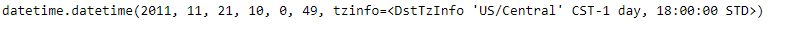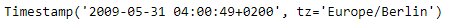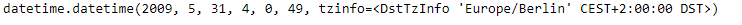# Python | Pandas Timestamp.to_pydatetime

Python is a great language for doing data analysis, primarily because of the fantastic ecosystem of data-centric python packages. Pandas is one of those packages and makes importing and analyzing data much easier.

Pandas` Timestamp.to_pydatetime()` function convert a Timestamp object to a native Python datetime object.

Syntax :Timestamp.to_pydatetime()

Parameters :

warn : boolean

Return : datetime object

Example #1: Use `Timestamp.to_pydatetime()` function to convert the given Timestamp to a native python datetime object.

 `# importing pandas as pd ` `import` `pandas as pd ` ` `  `# Create the Timestamp object ` `ts ``=` `pd.Timestamp(year ``=` `2011``,  month ``=` `11``, day ``=` `21``,  ` `                  ``hour ``=` `10``, second ``=` `49``, tz ``=` `'US/Central'``)  ` ` `  `# Print the Timestamp object ` `print``(ts) `

Output :Now we will use the `Timestamp.to_pydatetime()` function to convert the given Timestamp to python’s datetime object.

 `# convert to datetime ` `ts.to_pydatetime() `

Output :As we can see in the output, the `Timestamp.to_pydatetime()` function has returned a native python datetime object constructed from the given Timestamp object.

Example #2: Use `Timestamp.to_pydatetime()` function to convert the given Timestamp to a native python datetime object.

 `# importing pandas as pd ` `import` `pandas as pd ` ` `  `# Create the Timestamp object ` `ts ``=` `pd.Timestamp(year ``=` `2009``, month ``=` `5``, day ``=` `31``,  ` `                  ``hour ``=` `4``, second ``=` `49``, tz ``=` `'Europe/Berlin'``) ` ` `  `# Print the Timestamp object ` `print``(ts) `

Output :Now we will use the `Timestamp.to_pydatetime()` function to convert the given Timestamp to python’s datetime object.

 `# convert to datetime ` `ts.to_pydatetime() `

Output :As we can see in the output, the `Timestamp.to_pydatetime()` function has returned a native python datetime object constructed from the given Timestamp object.

My Personal Notes arrow_drop_upCheck out this Author's contributed articles.

If you like GeeksforGeeks and would like to contribute, you can also write an article using contribute.geeksforgeeks.org or mail your article to contribute@geeksforgeeks.org. See your article appearing on the GeeksforGeeks main page and help other Geeks.

Please Improve this article if you find anything incorrect by clicking on the "Improve Article" button below.

Article Tags :

Be the First to upvote.

Please write to us at contribute@geeksforgeeks.org to report any issue with the above content.